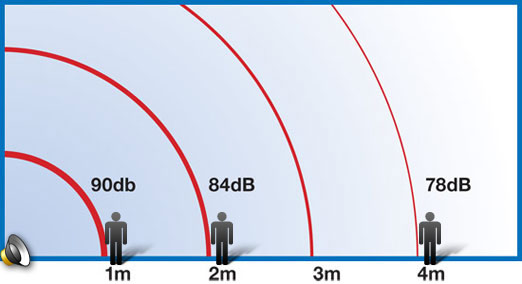# Inverse Square Law

Enter the following values and then press "Calculate" to determine the sound pressure level at the new distance from the source.

• UOM (ft, yd, m, etc.)
• dB SPL
• UOM (ft, yd, m, etc.)

## Results

SOUND LEVEL AT NEW dB

Sound pressure level - SPL decreases 6.02 dB as a listener's distance doubles from the sound source. This number is commonly rounded down to 6 dB. The opposite is true as the listener halves the distance to the source, so that the SPL decreases or increases by 6 dB with every distance doubling or halving, respectively. The Inverse Square Law is used to calculate the change in SPL with distance, and is useful in predicting the sound pressure level a listener will experience as speaker placement and sound levels are considered.• LN = LR - 20 log (DN/DR)
• DR = reference distance
• DN = new distance
• LR = SPL at reference distance
• LN = SPL at new distance